# MATHEMATICS CORE PAPER 1 END OF TERM 1 YEAR 11 DECEMBER 2021

Created
Best for live in-class or video conferencing lessonsStart teacher-led lesson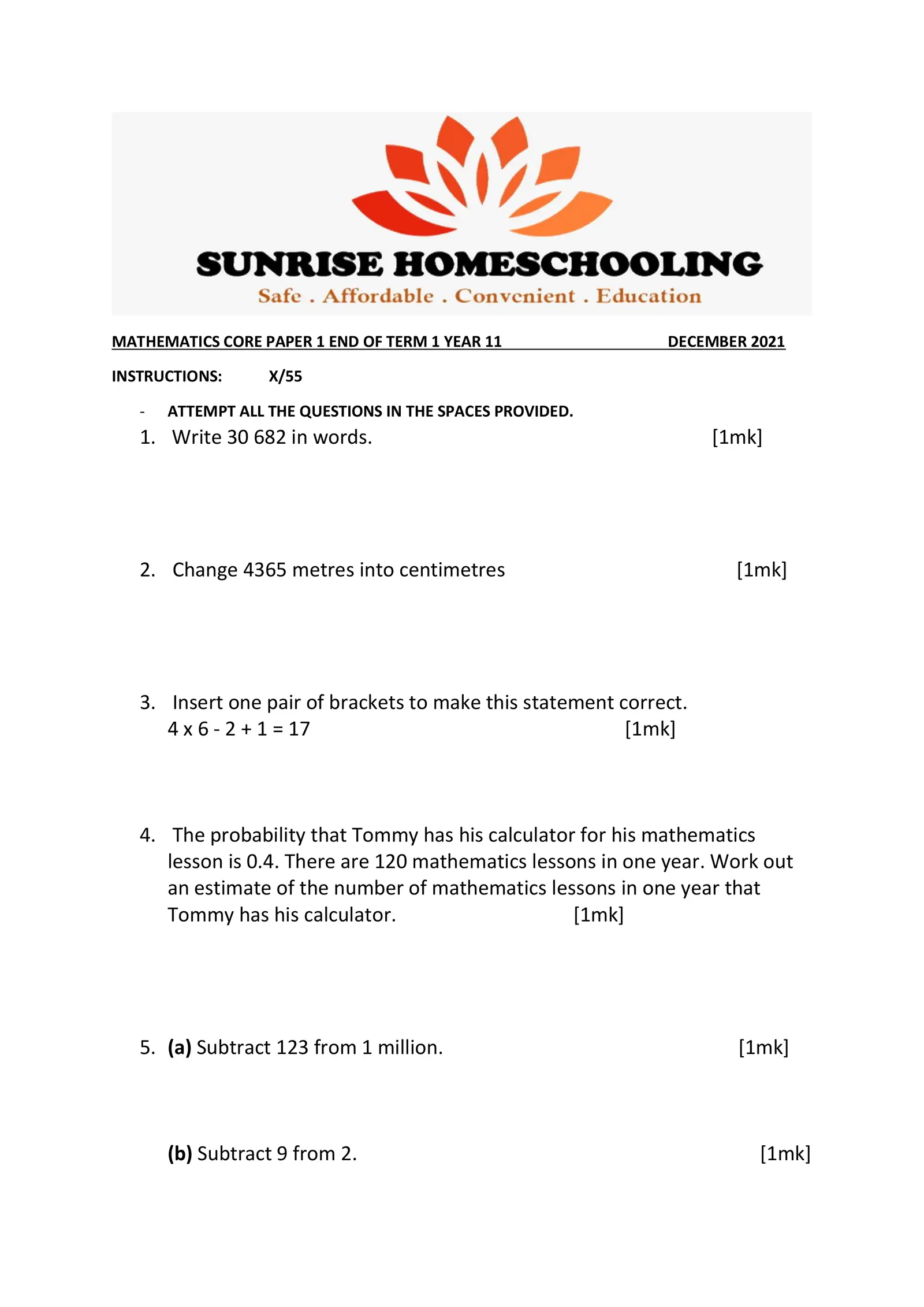MATHEMATICS CORE PAPER 1 END OF TERM 1 YEAR 11 DECEMBER 2021 INSTRUCTIONS: X/55 - ATTEMPT ALL THE QUESTIONS IN THE SPACES PROVIDED. 1. Write 30 682 in words. [1mk] 2. Change 4365 metres into centimetres [1mk] 3. Insert one pair of brackets to make this statement correct. 4 x 6 - 2 + 1 = 17 [1mk] 4. The probability that Tommy has his calculator for his mathematics lesson is 0.4. There are 120 mathematics lessons in one year. Work out an estimate of the number of mathematics lessons in one year that Tommy has his calculator. [1mk] 5. (a) Subtract 123 from 1 million. [1mk] (b) Subtract 9 from 2. [1mk]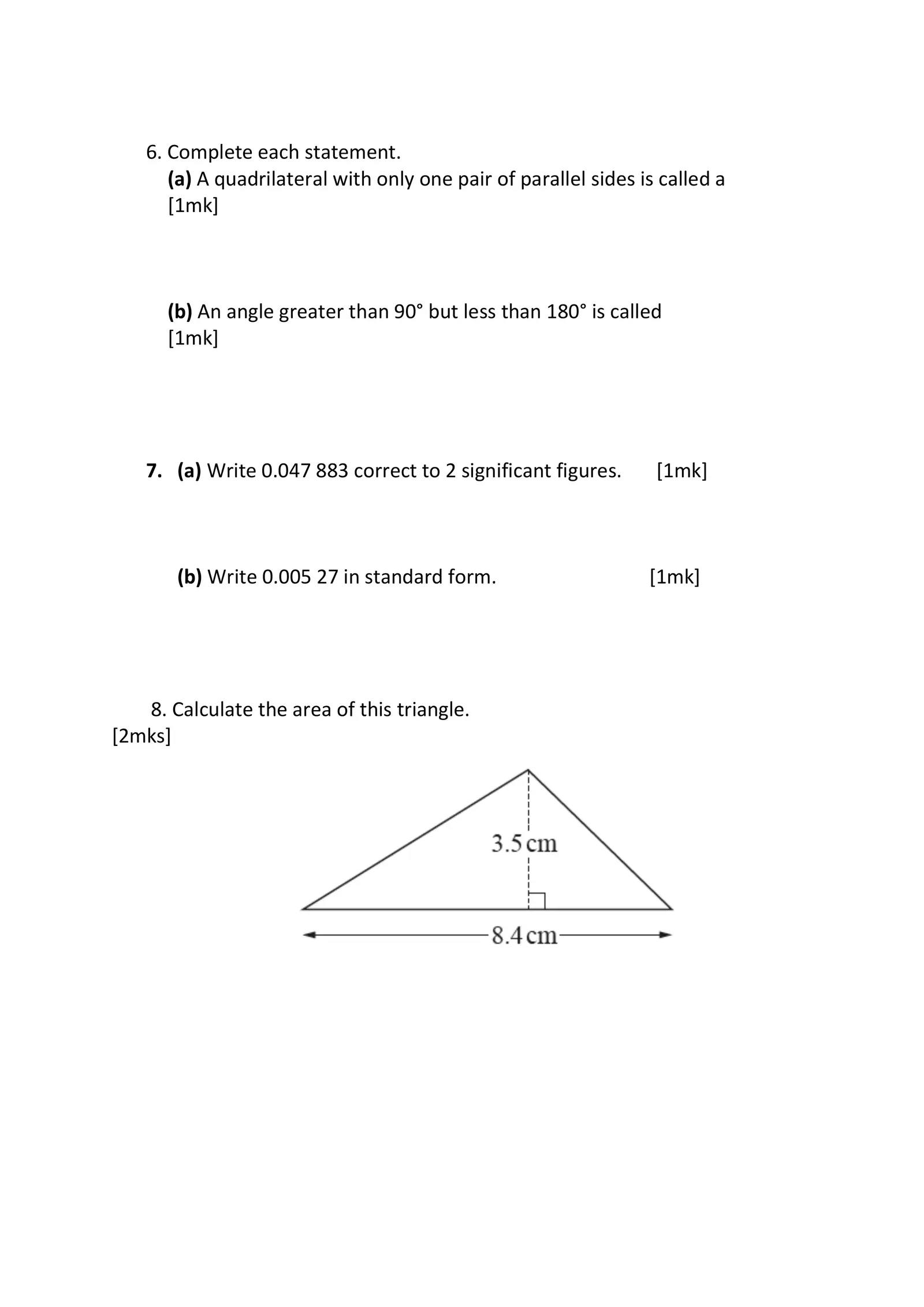6. Complete each statement. (a) A quadrilateral with only one pair of parallel sides is called a [1mk] (b) An angle greater than 90° but less than 180° is called [1mk] 7. (a) Write 0.047 883 correct to 2 significant figures. [1mk] (b) Write 0.005 27 in standard form. [1mk] 8. Calculate the area of this triangle. [2mks]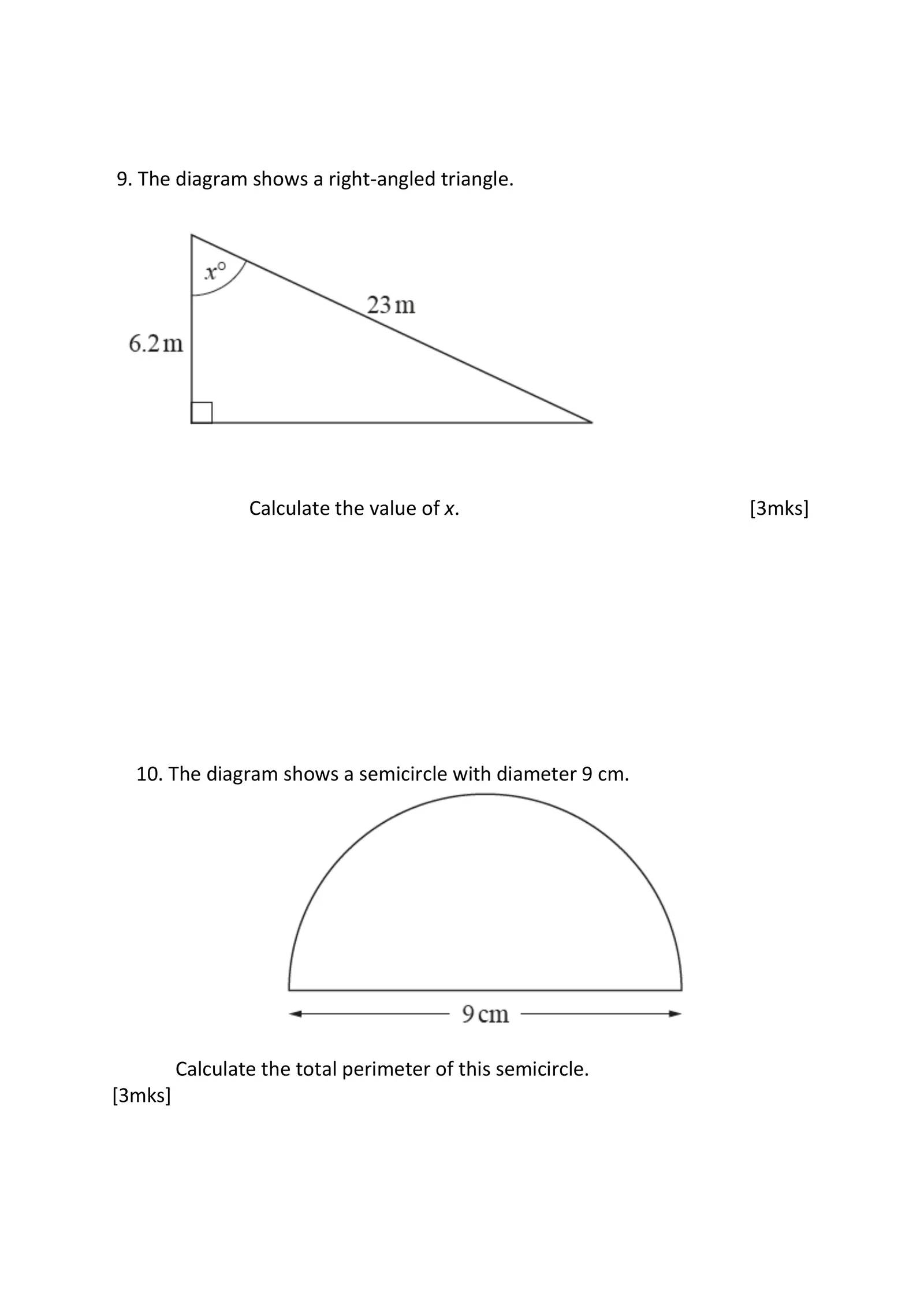9. The diagram shows a right-angled triangle. Calculate the value of x. [3mks] 10. The diagram shows a semicircle with diameter 9 cm. Calculate the total perimeter of this semicircle. [3mks]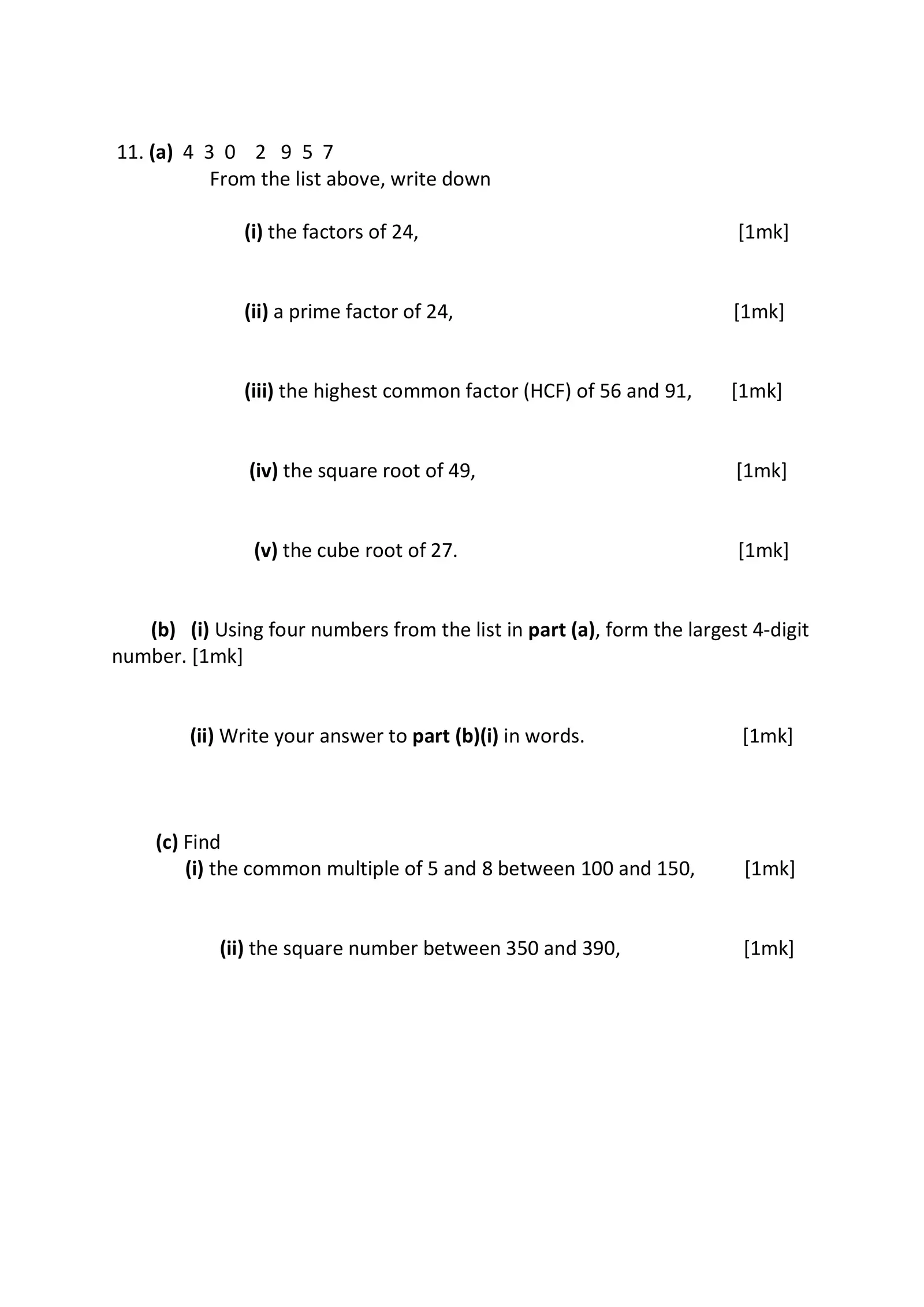11. (a) 4 3 0 2 9 5 7 From the list above, write down (i) the factors of 24, [1mk] (ii) a prime factor of 24, [1mk] (iii) the highest common factor (HCF) of 56 and 91, [1mk] (iv) the square root of 49, [1mk] (v) the cube root of 27. [1mk] (b) (i) Using four numbers from the list in part (a), form the largest 4-digit number. [1mk] (ii) Write your answer to part (b)(i) in words. [1mk] (c) Find (i) the common multiple of 5 and 8 between 100 and 150, [1mk] (ii) the square number between 350 and 390, [1mk]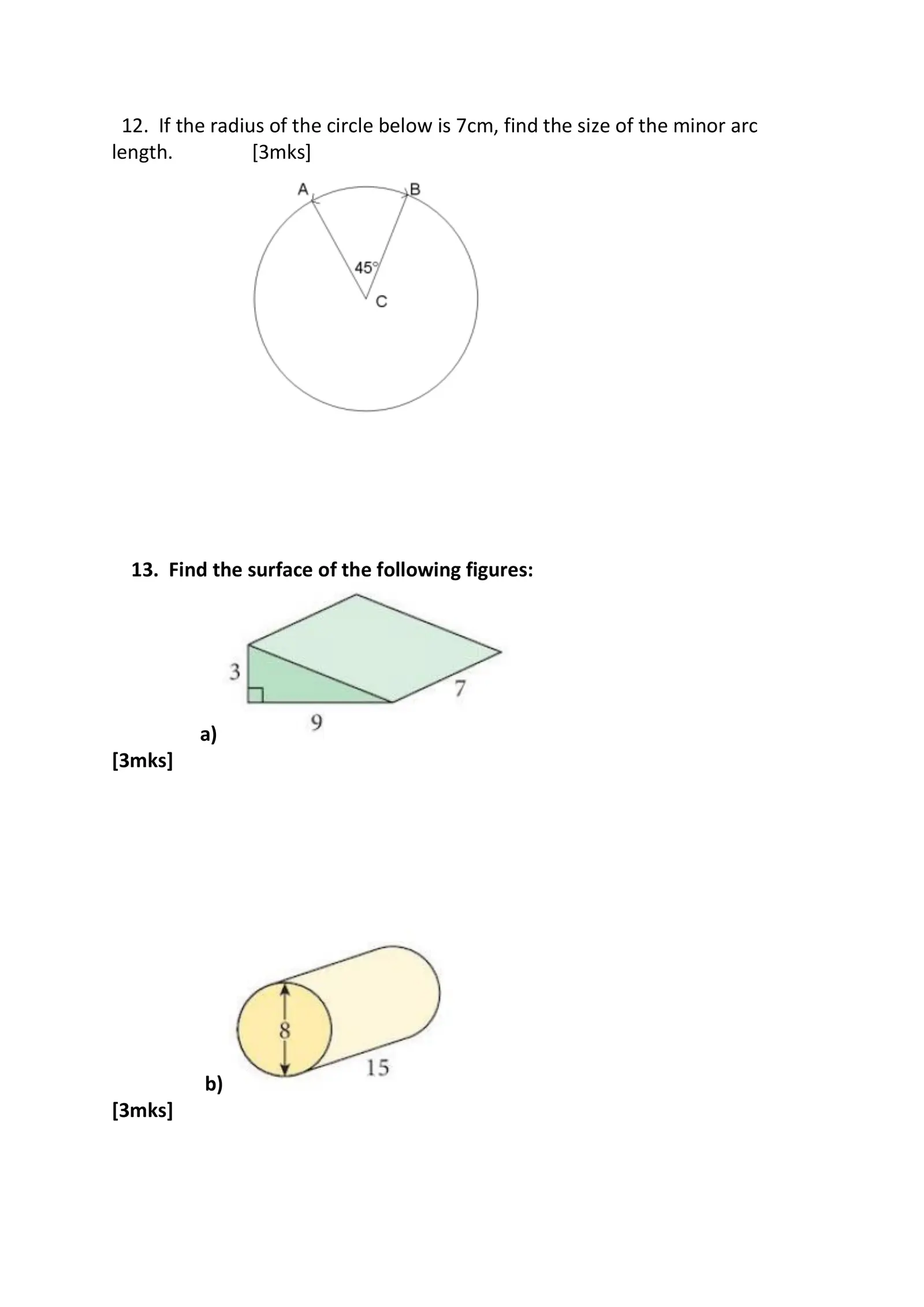12. If the radius of the circle below is 7cm, find the size of the minor arc length. [3mks] 13. Find the surface of the following figures: a) [3mks] b) [3mks]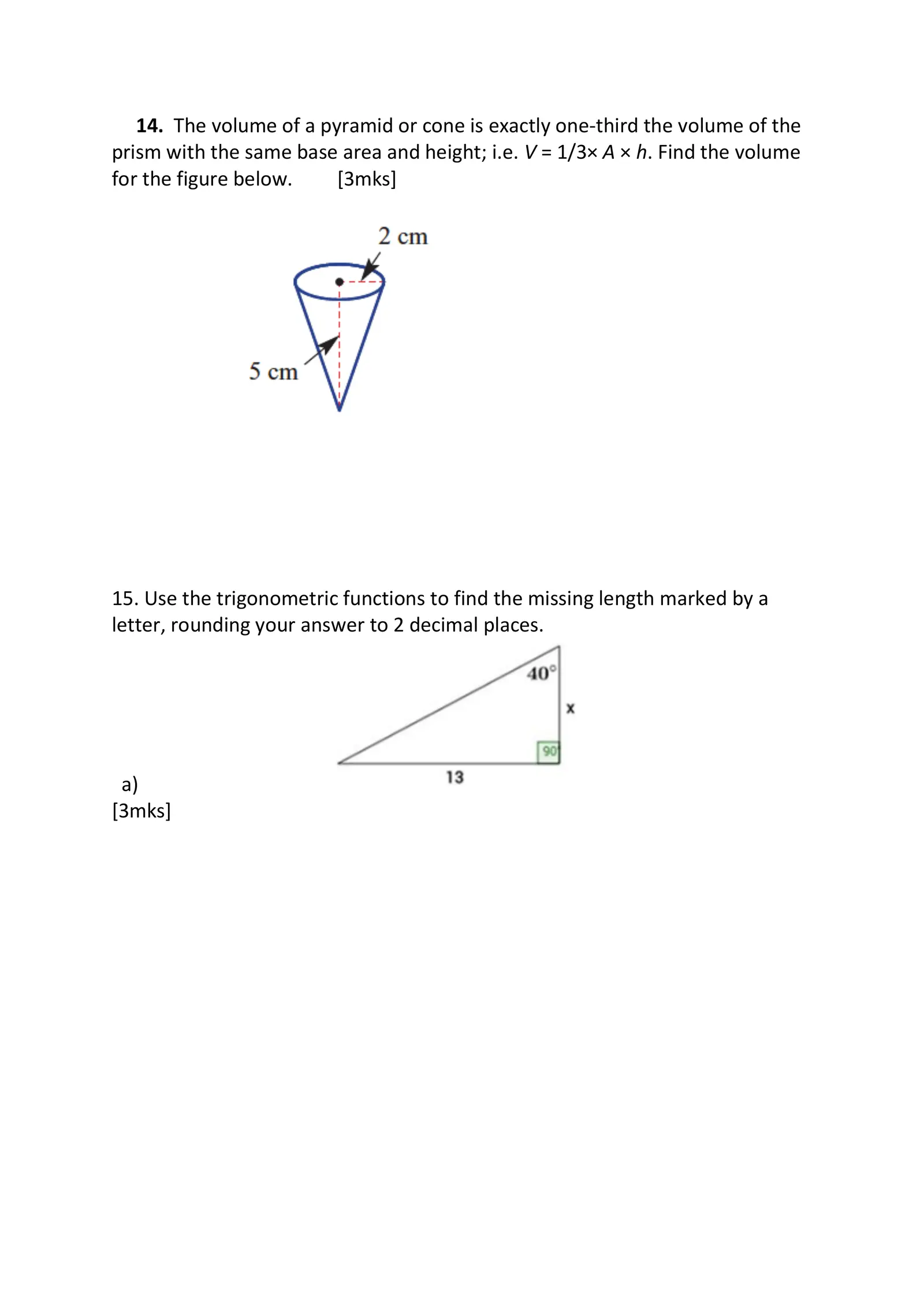14. The volume of a pyramid or cone is exactly one-third the volume of the prism with the same base area and height; i.e. V = 1/3× A × h. Find the volume for the figure below. [3mks] 15. Use the trigonometric functions to find the missing length marked by a letter, rounding your answer to 2 decimal places. a) [3mks]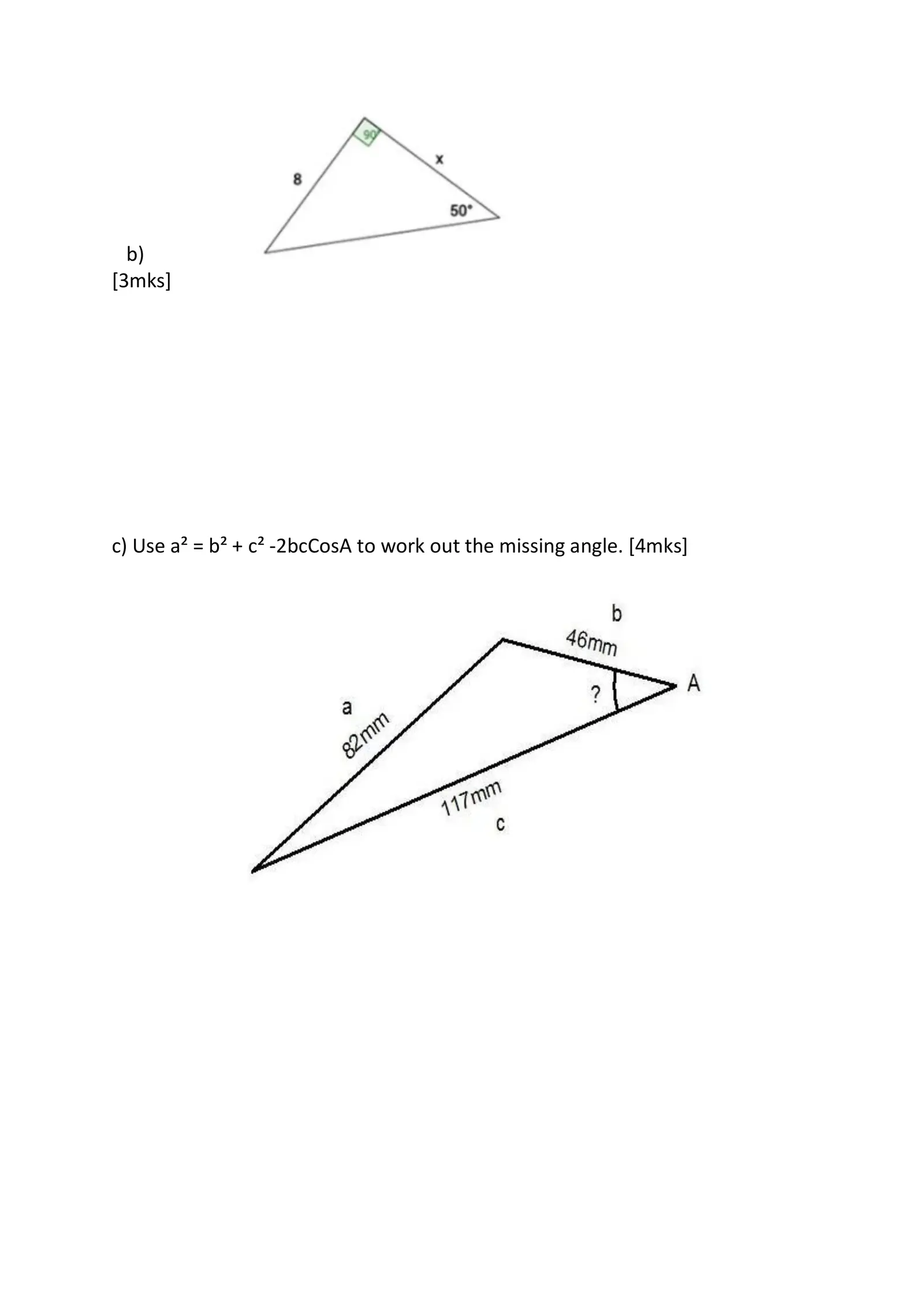b) [3mks] c) Use a² = b² + c² -2bcCosA to work out the missing angle. [4mks]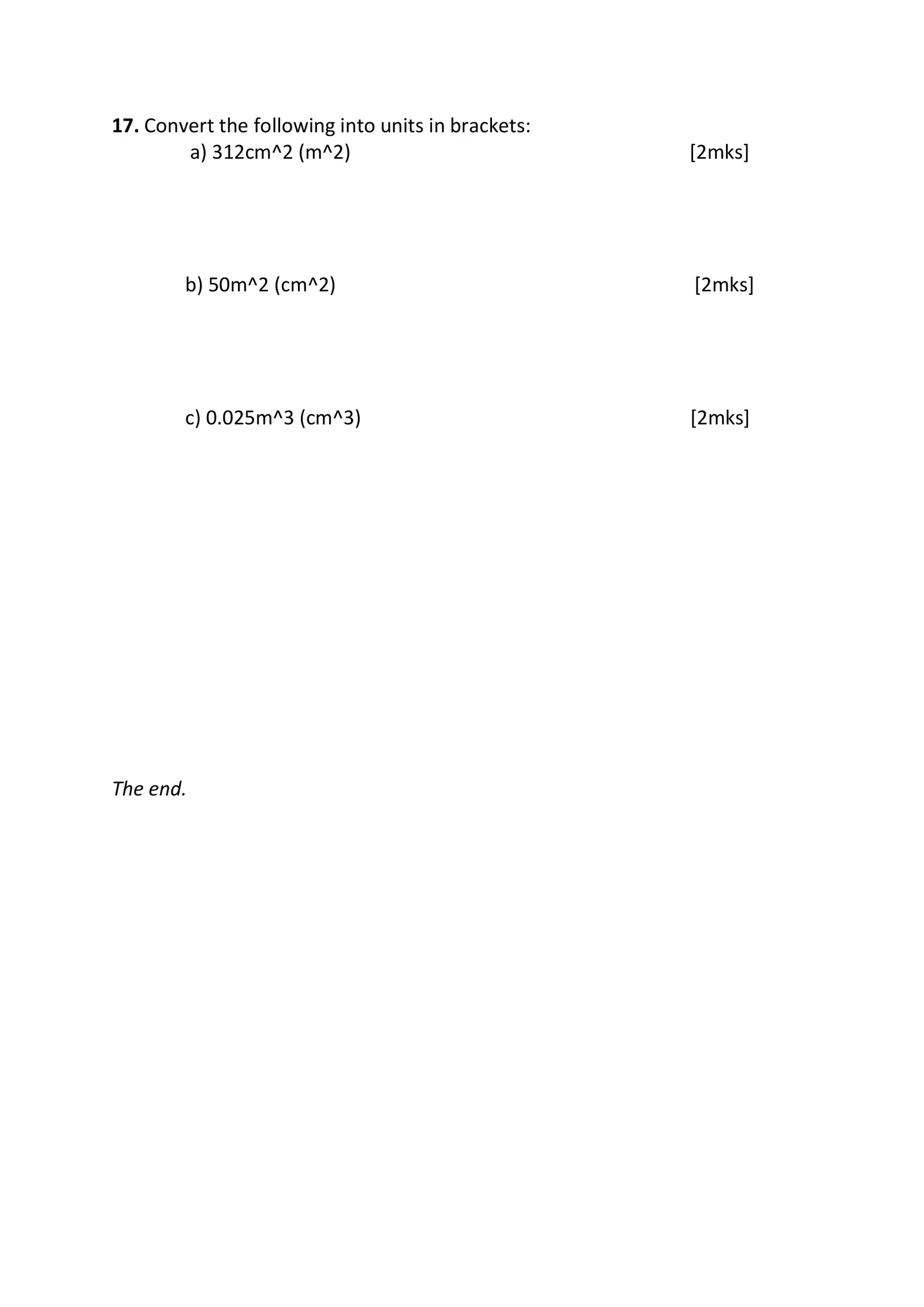17. Convert the following into units in brackets: a) 312cm^2 (m^2) [2mks] b) 50m^2 (cm^2) [2mks] c) 0.025m^3 (cm^3) [2mks] The end.